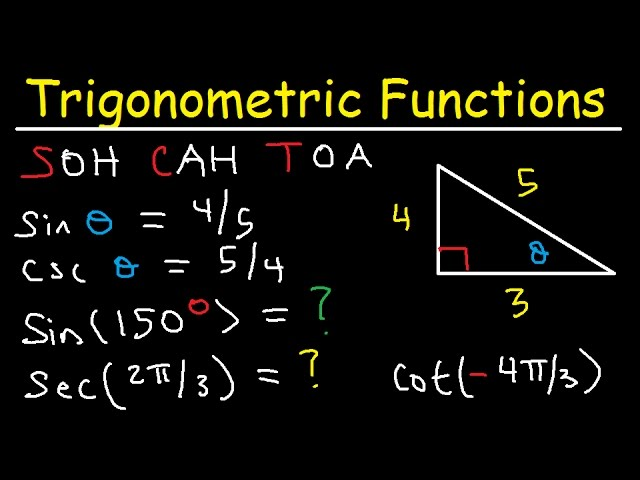# Relationship between Trigonometry and GeometryMath plays a significant role in the design and construction of buildings, highways, and bridges. Although we can solve many math problems with a computer or calculator, learning the basic principles used to solve the problems is necessary. This allows the relevant set-up, listing, and verification of the computations. Besides, manual calculations may be required when a computer is not available. Thus, different branches of mathematics help to solve various problems in our daily existence. More specifically, trigonometry and geometry are observed more often.

As we know, the word ‘trigonometry’ is derived from the Greek words ‘trigon’ and ‘metron’, which implies measuring the sides of a triangle. Geometry is a branch of mathematics that deals with different shapes and their properties. However, these two branches are related to each other when we deal with triangles. To recall, a triangle is a three-sided and two-dimensional figure. Based on the measures of sides and angles, we can define different types of triangles. Several trigonometric functions are defined based on the sides and angles of a right-angle triangle. Each of these functions possess a specific range and domain. These functions constitute a specific weightage for the students of secondary classes in their examinations.

Generally, we use geometric formulas, such as area and volume, to solve fundamental math problems, whereas trigonometry functions to work on such problems, where triangles and angles are involved. In the constructions of buildings, roadways and bridge designs, geometry plays a significant role in every phase, from the primary land survey to plan and structure to calculate contractor payments. The construction field is represented by different geometric elements such as points, lines, curves, arcs, circles, ovals, polygons and other defined geometric shapes during site surveys.

Trigonometry is mainly used to solve problems involving heights and distances, whether each of the situations will be represented pictorially. Each of these diagrams involves at least one right-angled triangle so that we can find the required parameters using suitable functions and identities of trigonometry. Also, in the construction of geometric figures such as triangles, we use trigonometry. When we deal with the construction of an ambiguous case that means oblique triangles, we must use the law of sines to complete the construction. In this way, trigonometry and geometry are related to each other while solving different maths problems. This relationship helps us in solving complex problems in geometry. All these applications will be introduced with the students in their secondary and higher education classes.

We can explain the relationship between geometry and trigonometry with the help of some corresponding theorems. However, they seem to be different in geometry than in trigonometry. For example, there are three congruence theorems in geometry, namely side-side-side (SSS), side-angle-side (SAS), and angle-side-angle(ASA). Those, along with a part of geometry and algebra, provide techniques to solve oblique triangles (triangles that are not right triangles). The specific computations are expressed in the law of cosines and sines. The important point is that when we deal with obtuse triangles, the trigonometry functions for obtuse angles are defined the way they are.